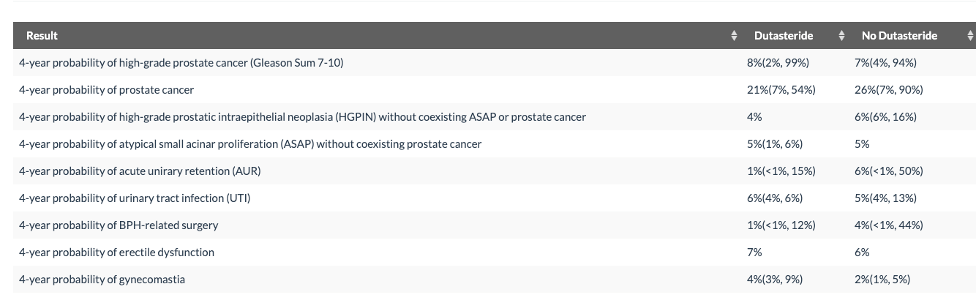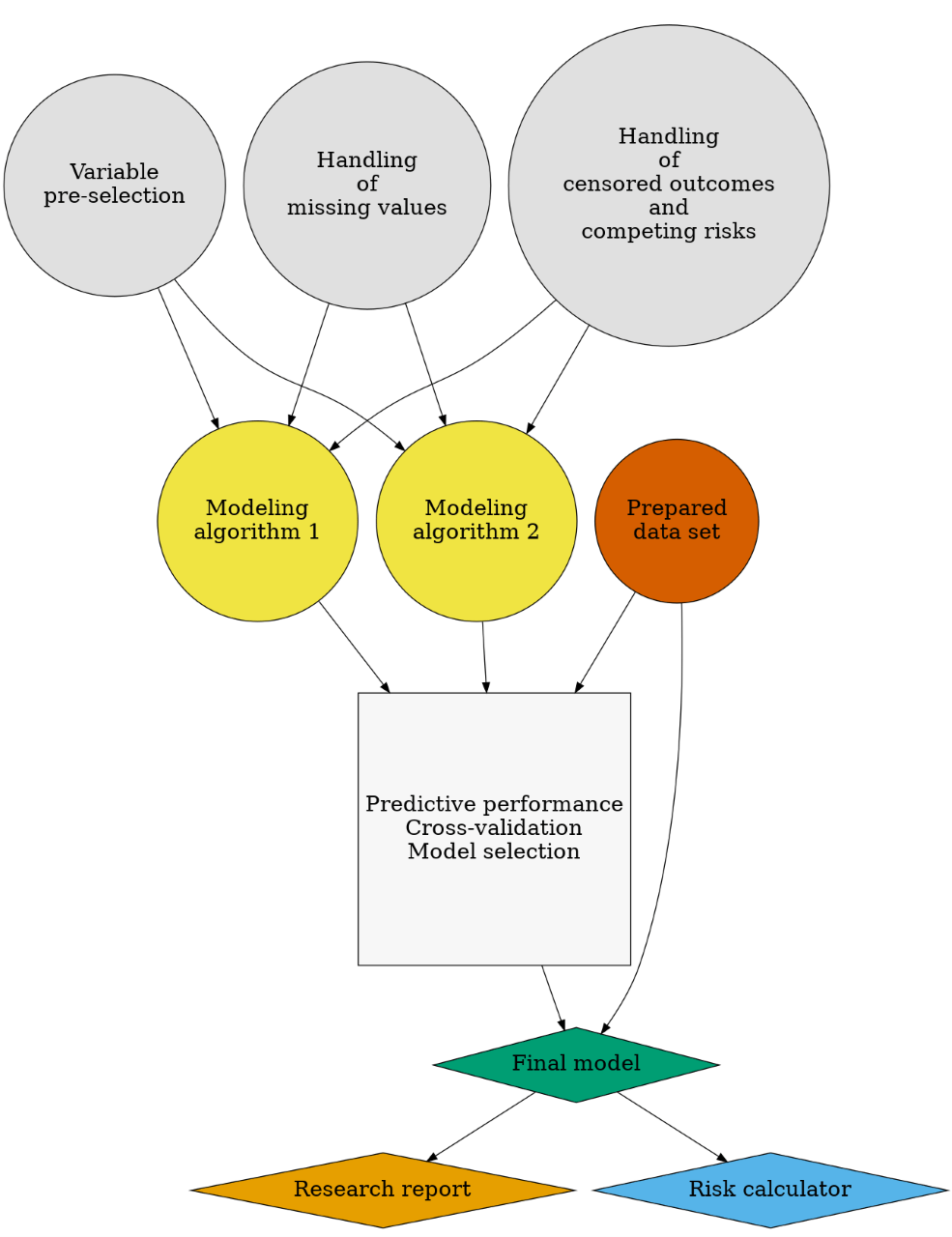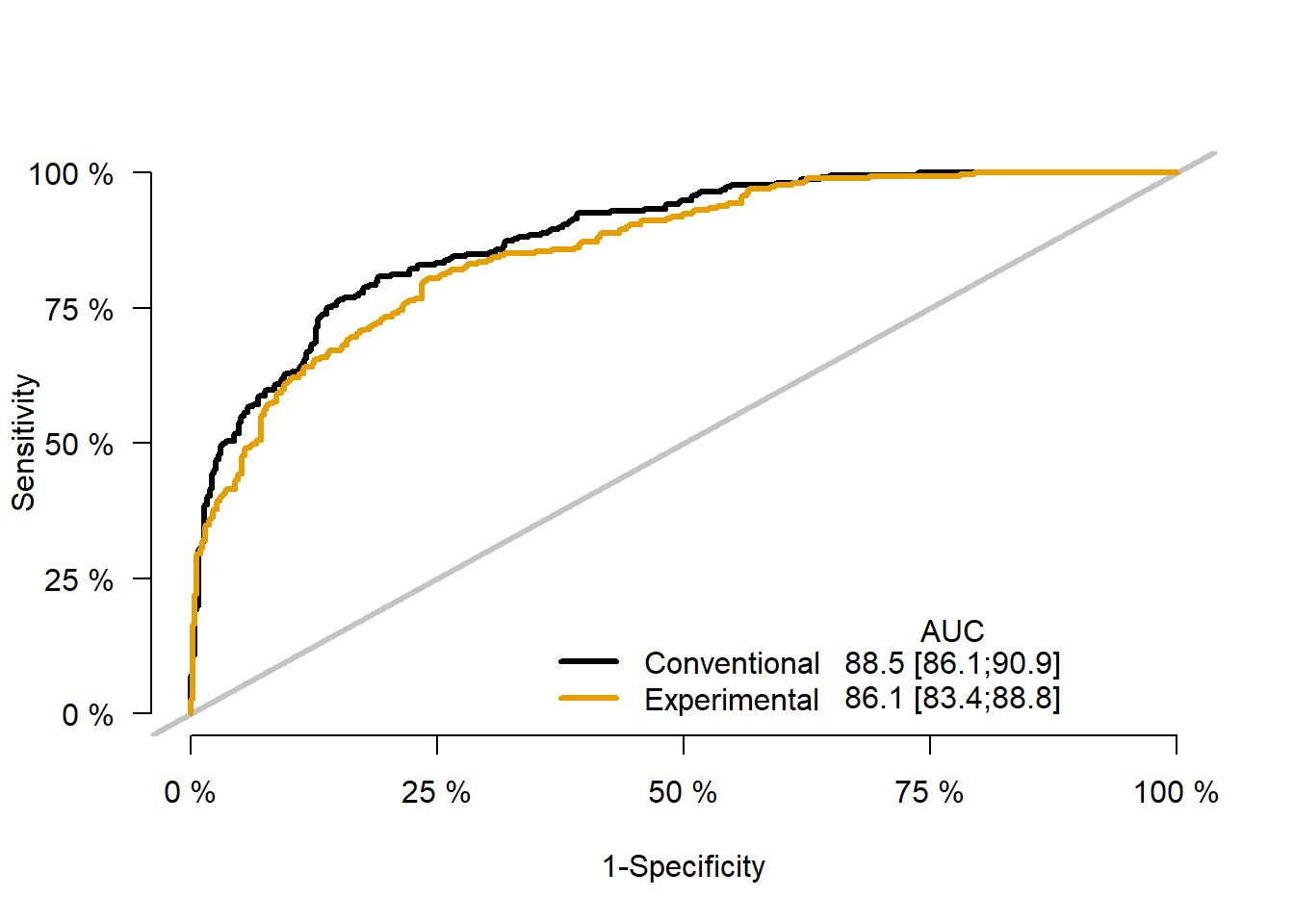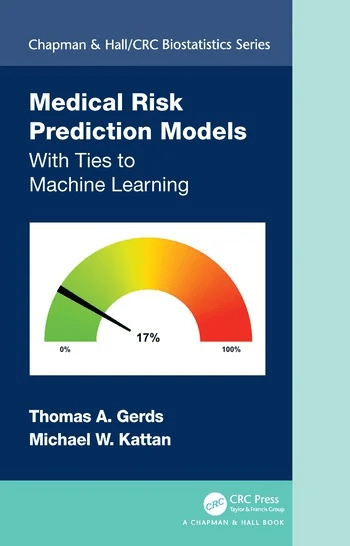Want to share your content on R-bloggers? click here if you have a blog, or here if you don't.

We first started making risk calculators with the website, http://nomograms.org. There are several here, and they are patient friendly. Later, we launched rcalc.ccf.org, which has many more risk calculators, although the intended audience is the clinician. It is considerably more expensive and time-consuming to make these patient friendly. There were many obstacles to overcome in making these websites. Here is an example (Figure 1), from prostate cancer research where our calculator assists with an optional treatment by showing the 4-year risks of several outcomes for both options:Figure 1: Example of what risk calculator shows to the user. Note that there are two models, one for Dutasteride and one for No Dutasteride with different sets of predictor variables. For some outcomes there is an interval on the Dutasteride but no interval on the No Dutasteride predicted risk. This happens when a variable which is only included in the model Dutasteride model has a missing value.

If the user were to provide some of the values of the predictor variables that are currently missing, his 4-year risk of high-grade prostate cancer (which is currently at 17% on Dutasteride) might be as low as 7% or as high as 49%. Clearly, obtaining missing values to resolve this uncertainty should be pursued. The missing values will have less of an impact on his risk for a urinary tract infection.

The need for a risk calculator begins with the subject matter expert. He or she needs to explain the decision-making context and the tradeoffs involved. Important questions to discuss are:

• Who will use the calculator and for what purpose?
• If a medical decision is based on the predicted risks: What are the benefits and harms?
• What types of outcome are predicted? (Uncensored, censored, censored with competing risk?)
• What are the important predictors of these outcomes? (Missing values?)

Questions like those are best answered in collaboration with the subject matter expert. We have learned that making a risk calculator without involving a subject matter expert leads to a risk calculator that on one uses.

The next steps are:

1. to evaluate and prepare the data sources
2. to select candidate variables
3. to establish a modeling strat (see Figure 2)
4. to report the final model in form of a shiny appFigure 2: Flowchart: prediction modeling strategy

Our R-package riskRegression provides implementations of modeling algorithms for censored data with competing risks and the cross-validation performance as described here online. Here is sample code:

```# sample R-code
library(riskRegression)
library(survival)
library(randomForestSRC)
# simulate competing risk data
set.seed(9)
train.data <- sampleData(n=472)
test.data <- sampleData(n=811)
# combined cause-specific Cox regression
fit1 <- CSC(Hist(time,event)~X1+X2+X3+X4+X5+X6+X7+X8+X9, data=train.data, cause=1)
# random forest
set.seed(1972)
fit2 <- rfsrc(Surv(time,event)~X1+X2+X3+X4+X5+X6+X7+X8+X9,data=train.data)
# prediction performance in independent test data
# could also do cross-validation of training data via argument split.method
x <- Score(list("Conventional"=fit1,"Experimental"=fit2),
data=test.data,
formula=Hist(time,event)~1,
cause=1,
times=c(1,5),
summary=c("risks","IPA"),
plots=c("cali","roc"))
plotROC(x,times=5)````plotCalibration(x,times=5)`The details of making an online risk calculator, how we deal with the many issues that arise, and many R-code examples, are described in our new book, available hereThis post is based on:

Kattan, M. W., & Gerds, T. A. (2020). A framework for the evaluation of statistical prediction models. Chest, 158(1), S29-S38. link